Chapter 4. Chemical Reactions and Equations

# Oxidation-Reduction Reactions

Learning Objectives

1. Define oxidation and reduction.
2. Assign oxidation numbers to atoms in simple compounds.
3. Recognize a reaction as an oxidation-reduction reaction.

Consider this chemical reaction:

2Na(s) + Cl2(g) → 2NaCl

The reactants are elements, and it is assumed that they are electrically neutral; they have the same number of electrons as protons. The product, however, is ionic; it is composed of Na+ and Cl ions. Somehow, the individual sodium atoms as reactants had to lose an electron to make the Na+ ion, while the chlorine atoms as reactants had to each gain an electron to become the Cl ion. This reaction involves the transfer of electrons between atoms.

In reality, electrons are lost by some atoms and gained by other atoms simultaneously. However, mentally we can separate the two processes.  is defined as the loss of one or more electrons by an atom.  is defined as the gain of one or more electrons by an atom. In reality, oxidation and reduction always occur together; it is only mentally that we can separate them. Chemical reactions that involve the transfer of electrons are called .

Redox reactions require that we keep track of the electrons assigned to each atom in a chemical reaction. How do we do that? We use an artificial count called the  to keep track of electrons in atoms. Oxidation numbers are assigned to atoms based on a series of rules. Oxidation numbers are not necessarily equal to the charge on the atom; we must keep the concepts of charge and oxidation numbers separate.

The rules for assigning oxidation numbers to atoms are as follows:

1. Atoms in their elemental state are assigned an oxidation number of 0.
2. Atoms in monatomic (i.e., one-atom) ions are assigned an oxidation number equal to their charge. Oxidation numbers are usually written with the sign first, then the magnitude, which differentiates them from charges.
3. In compounds, fluorine is assigned a −1 oxidation number; oxygen is usually assigned a −2 oxidation number (except in peroxide compounds [where it is −1] and in binary compounds with fluorine [where it is positive]); and hydrogen is usually assigned a +1 oxidation number (except when it exists as the hydride ion, H, in which case rule 2 prevails).
4. In compounds, all other atoms are assigned an oxidation number so that the sum of the oxidation numbers on all the atoms in the species equals the charge on the species (which is zero if the species is neutral).

Let us work through a few examples for practice. In H2, both hydrogen atoms have an oxidation number of 0, by rule 1. In NaCl, sodium has an oxidation number of +1, while chlorine has an oxidation number of −1, by rule 2. In H2O, the hydrogen atoms each have an oxidation number of +1, while the oxygen has an oxidation number of −2, even though hydrogen and oxygen do not exist as ions in this compound as per rule 3. By contrast, by rule 3 in hydrogen peroxide (H2O2), each hydrogen atom has an oxidation number of +1, while each oxygen atom has an oxidation number of −1. We can use rule 4 to determine oxidation numbers for the atoms in SO2. Each oxygen atom has an oxidation number of −2; for the sum of the oxidation numbers to equal the charge on the species (which is zero), the sulfur atom is assigned an oxidation number of +4. Does this mean that the sulfur atom has a 4+ charge on it? No, it only means that the sulfur atom is assigned a +4 oxidation number by our rules of apportioning electrons among the atoms in a compound.

Example 4.11

# Problems

Assign oxidation numbers to the atoms in each substance.

1. Br2
2. SiO2
3. Ba(NO3)2

## Solutions

1. Br2 is the elemental form of bromine. Therefore, by rule 1, each atom has an oxidation number of 0.
2. By rule 3, oxygen is normally assigned an oxidation number of −2. For the sum of the oxidation numbers to equal the charge on the species (which is zero), the silicon atom is assigned an oxidation number of +4.
3. The compound barium nitrate can be separated into two parts: the Ba2+ ion and the nitrate ion. Considering these separately, the Ba2+ ion has an oxidation number of +2 by rule 2. Now consider the NO3 ion. Oxygen is assigned an oxidation number of −2, and there are three oxygens. According to rule 4, the sum of the oxidation number on all atoms must equal the charge on the species, so we have the simple algebraic equation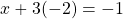, where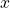is the oxidation number of the nitrogen atom and −1 represents the charge on the species. Evaluating,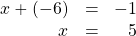Thus, the oxidation number on the N atom in the nitrate ion is +5.

# Test Yourself

Assign oxidation numbers to the atoms in H3PO4.

H = +1, O = −2, P = +5

All redox reactions occur with a simultaneous change in the oxidation numbers of some atoms. At least two elements must change their oxidation numbers. When an oxidation number of an atom is increased in the course of a redox reaction, that atom is being oxidized. When an oxidation number of an atom is decreased in the course of a redox reaction, that atom is being reduced. Oxidation and reduction are thus also defined in terms of increasing or decreasing oxidation numbers, respectively.

Example 4.12

# Problem

Identify what is being oxidized and reduced in this redox equation:

2Na + Cl2 → 2NaCl

## Solution

Consider the reactants. Because both reactants are the elemental forms of their atoms, the Na and Cl atoms as reactants have oxidation numbers of 0. In the ionic product, the sodium ions have an oxidation number of +1, while the chloride ions have an oxidation number of −1: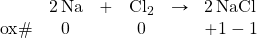We note that the sodium is increasing its oxidation number from 0 to +1, so it is being oxidized; chlorine is decreasing its oxidation number from 0 to −1, so it is being reduced.

Because oxidation numbers are changing, this is a redox reaction. Note that the total number of electrons lost by the sodium (two, one lost from each atom) is gained by the chlorine atoms (two, one gained for each atom).

# Test Yourself

Identify what is being oxidized and reduced in this redox equation.

C + O2 → CO2

C is being oxidized from 0 to +4; O is being reduced from 0 to −2.

In this introduction to oxidation-reduction reactions, we are using straightforward examples. However, oxidation reactions can become quite complex; the following equation represents a redox reaction: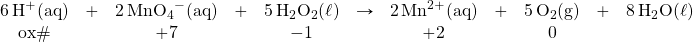To demonstrate that this is a redox reaction, the oxidation numbers of the species being oxidized and reduced are listed; can you determine what is being oxidized and what is being reduced? This is also an example of a net ionic reaction; spectator ions that do not change oxidation numbers are not displayed in the equation.

# Food and Drink App: Acids in Foods

Many foods and beverages contain acids. Acids impart a sour note to the taste of foods, which may add some pleasantness to the food. For example, orange juice contains citric acid, H3C6H5O7. Note how this formula shows hydrogen atoms in two places; the first hydrogen atoms written are the hydrogen atoms that can form H+ ions, while the second hydrogen atoms written are part of the citrate ion, C6H5O73−. Lemons and limes contain much more citric acid—about 60 times as much—which accounts for these citrus fruits being more sour than most oranges. Vinegar is essentially a ~5% solution of acetic acid (HC2H3O2) in water. Apples contain malic acid (H2C4H4O5; the name malic acid comes from the apple’s botanical genus name, malus), while lactic acid (HC3H5O3) is found in wine and sour milk products, such as yogurt and some cottage cheeses.

Table 4.2 “Various Acids Found in Food and Beverages” lists some acids found in foods, either naturally or as an additive. Frequently, the salts of acid anions are used as additives, such as monosodium glutamate (MSG), which is the sodium salt derived from glutamic acid. As you read the list, you should come to the inescapable conclusion that it is impossible to avoid acids in food and beverages.

Table 4.2 Various Acids Found in Food and Beverages
Acid Name Acid Formula Use and Appearance
acetic acid HC2H3O2 flavouring; found in vinegar
adipic acid H2C6H8O4 flavouring; found in processed foods and some antacids
alginic acid various thickener; found in drinks, ice cream, and weight loss products
ascorbic acid HC6H7O6 antioxidant, also known as vitamin C; found in fruits and vegetables
benzoic acid HC6H5CO2 preservative; found in processed foods
citric acid H3C6H5O7 flavouring; found in citrus fruits
dehydroacetic acid HC8H7O4 preservative, especially for strawberries and squash
erythrobic acid HC6H7O6 antioxidant; found in processed foods
fatty acids various thickener and emulsifier; found in processed foods
fumaric acid H2C4H2O4 flavouring; acid reactant in some baking powders
glutamic acid H2C5H7NO4 flavouring; found in processed foods and in tomatoes, some cheeses, and soy products
lactic acid HC3H5O3 flavouring; found in wine, yogurt, cottage cheese, and other sour milk products
malic acid H2C4H4O5 flavouring; found in apples and unripe fruit
phosphoric acid H3PO4 flavouring; found in some colas
propionic acid HC3H5O2 preservative; found in baked goods
sorbic acid HC6H7O2 preservative; found in processed foods
stearic acid HC18H35O2 anticaking agent; found in hard candies
succinic acid H2C4H4O4 flavouring; found in wine and beer
tartaric acid H2C4H4O6 flavouring; found in grapes, bananas, and tamarinds

Key Takeaways

• Oxidation-reduction (redox) reactions involve the transfer of electrons from one atom to another.
• Oxidation numbers are used to keep track of electrons in atoms.
• There are rules for assigning oxidation numbers to atoms.
• Oxidation is an increase of oxidation number (a loss of electrons); reduction is a decrease in oxidation number (a gain of electrons).

Exercises

# Questions

1. Is the reaction 2K(s) + Br2(ℓ) → 2KBr(s) an oxidation-reduction reaction? Explain your answer.
2. Is the reaction NaCl(aq) + AgNO3(aq) → NaNO3(aq) + AgCl(s) an oxidation-reduction reaction? Explain your answer.
3. In the reaction 2Ca(s) + O2(g) → 2CaO, indicate what has lost electrons and what has gained electrons.
4. In the reaction 16Fe(s) + 3S8(s) → 8Fe2S3(s), indicate what has lost electrons and what has gained electrons.
5. In the reaction 2Li(s) + O2(g) → Li2O2(s), indicate what has been oxidized and what has been reduced.
6. In the reaction 2Ni(s) + 3I2(s) → 2NiI3(s), indicate what has been oxidized and what has been reduced.
7. What are two different definitions of oxidation?
8. What are two different definitions of reduction?
9. Assign oxidation numbers to each atom in each substance.
1. P4
2. SO2
3. SO22−
4. Ca(NO3)2
10. Assign oxidation numbers to each atom in each substance.
1. PF5
2. (NH4)2S
3. Hg
4. Li2O2 (lithium peroxide)
11. Assign oxidation numbers to each atom in each substance.
1. CO
2. CO2
3. NiCl2
4. NiCl3
12. Assign oxidation numbers to each atom in each substance.
1. NaH (sodium hydride)
2. NO2
3. NO2
4. AgNO3
13. Assign oxidation numbers to each atom in each substance.
1. CH2O
2. NH3
3. Rb2SO4
4. Zn(C2H3O2)2
14. Assign oxidation numbers to each atom in each substance.
1. C6H6
2. B(OH)3
3. Li2S
4. Au
15. Identify what is being oxidized and reduced in the redox equation 2NO + Cl2 → 2NOCl by assigning oxidation numbers to the atoms.
16. Identify what is being oxidized and reduced in the redox equation Fe + SO3 → FeSO3 by assigning oxidation numbers to the atoms.
17. Identify what is being oxidized and reduced in the redox equation 2KrF2 + 2H2O → 2Kr + 4HF + O2 by assigning oxidation numbers to the atoms.
18. Identify what is being oxidized and reduced in the redox equation SO3 + SCl2 → SOCl2 + SO2 by assigning oxidation numbers to the atoms.
19. Identify what is being oxidized and reduced in the redox equation 2K + MgCl2 → 2KCl + Mg by assigning oxidation numbers to the atoms.
20. Identify what is being oxidized and reduced in the redox equation C7H16 + 11O2 → 7CO2 + 8H2O by assigning oxidation numbers to the atoms.

1. Yes; both K and Br are changing oxidation numbers.
1. Ca has lost electrons, and O has gained electrons.
1. Li has been oxidized, and O has been reduced.
1. Loss of electrons; increase in oxidation number.
1. P: 0
2. S: +4; O: −2
3. S: +2; O: −2
4. Ca: 2+; N: +5; O: −2
1. C: +2; O: −2
2. C: +4; O: −2
3. Ni: +2; Cl: −1
4. Ni: +3; Cl: −1
1. C: 0; H: +1; O: −2
2. N: −3; H: +1
3. Rb: +1; S: +6; O: −2
4. Zn: +2; C: 0; H: +1; O: −2
1. N is being oxidized, and Cl is being reduced.
1. O is being oxidized, and Kr is being reduced.
1. K is being oxidized, and Mg is being reduced.